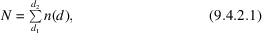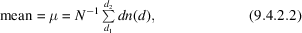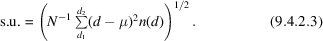International
Tables for
Crystallography
Volume C
Mathematical, physical and chemical tables
Edited by E. Prince

International Tables for Crystallography (2006). Vol. C, ch. 9.4, p. 778

## Section 9.4.2. The retrieval system

G. Bergerhoffa and K. Brandenburga

aInstitut für Anorganische Chemie der Universität Bonn, Gerhard-Domagkstrasse 1, D-53121 Bonn, Germany

### 9.4.2. The retrieval system

| top | pdf |

By means of the retrieval system CRYSTIN (Sievers & Hundt, 1987), sets of structures were selected and combined; these contained:

 (i) one ion in a specific oxidation state (if appropriate); (ii) the other ion of the pair in a specific oxidation state (if appropriate); (iii) structures determined at room temperature; (iv) structures determined at normal pressure; (v) no disordered structures; (vi) no solid solutions; (vii) no defect structures; (viii) structures for which atomic coordinates were given; (ix) only structures with R < 6% when there were many determinations.

For the M structures so defined, n interatomic distances were calculated for the atoms and ions in question. In so doing, all symmetrically equivalent and non-equivalent distances were taken into account. From these, a frequency distribution was calculated automatically. All distances d were collected into ranges of 2 pm. In (9.4.2.1)–(9.4.2.2), n(d) is the number of distances in the range with midpoint d,is the midpoint of the lowest range, andis the midpoint of the highest range selected for the calculation of the mean. The choice ofandis discussed in Section 9.4.3. The mean and the standard uncertainty (s.u.) of one set of distances were calculated by means of the equations### References

Sievers, R. & Hundt, R. (1987). Crystallographic information system CRYSTIN. In Crystallographic databases, edited by F. H. Allen, G. Bergerhoff & R. Sievers, pp. 210–221. Bonn/Cambridge/Chester: International Union of Crystallography.